# Circuits

In this article, the fundamentals of electric charge, current, potential difference, resistance and conservation of charge are discussed.

Electric Charge

Matter is made up of tiny particles called atoms. An atom consists of protons, neutrons and electrons. Normally, the number of protons and electrons are equal and the overall charge of an atom is zero. But, for certain atoms, electrons in the outer shell of the atom can be easily removed. An atom with such unbalanced positive charges becomes positively charged and is called a positive ion. Electric charge is measured in coulombs (C) and an electron consists of -1.6×10^19 C.

Electric Current

Figure 1 shows an electric circuit that consists of a battery, wire, switch and bulb. The wire and filament of the bulb are made of metal. Atoms of a metal have one or more electrons that are free to move. These electrons are called free electrons.Figure 1: Simple Electric Circuit

When the switch is closed, the electrons move from the negative terminal to the positive terminal, due to the energy from the battery, and the bulb glows. This flow of electrons is called a current. Benjamin Franklin thought electricity flows from a positively charged substance to a negatively charged substance. His idea is still used today and is known as the conventional current direction. Figure 2 shows the direction of electron flow and conventional current direction.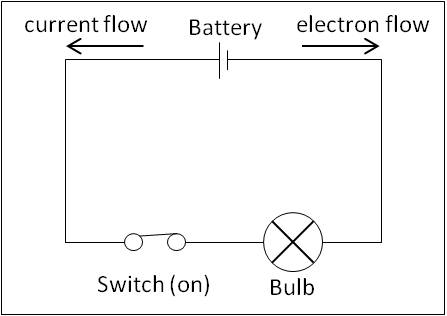Figure 2: Conventional Current Flow

The rate at which the electrons flow in a circuit is measured in amperes (A). One ampere of current is approximately equivalent to 6.24×10^18 electrons moving past a boundary in one second.

The amount of charge (Q) is determined by a steady current (I) flowing in the circuit for a time (t).

Q=I×t

Potential Difference

In Figure 1, to flow through the circuit, the electrons obtain energy from the battery. A potential difference is set up across the positive and negative terminal of the battery which causes the charges’ flow.

The potential difference between any two points in a circuit is the measure of work done by an electron to move from one point to another. Potential energy is measured in volts (V) and energy is measured in joules (J).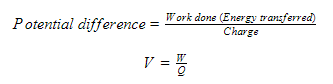One volt is the potential difference between two points when one joule of energy is transferred from electrical to other forms by one coulomb of electric charge passing from one point to another.

Resistance

Resistance is the measure of the difficulty of an electron to flow through the conductor. If the resistance is increased, electron flow decreases and hence the current decreases. When the resistance is lowered, the current increases as the electron flow increases. Resistance of a conductor is defined as the ratio of potential difference (V) across it to the current (I) flowing through it. The unit for resistance is the ohm (Ω).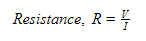Combining Resistors

Two or more resistors can be replaced by a single resistor that has an equivalent effect on the circuit. According to the connection between resistors, the equivalent resistance is calculated.

When the resistors are connected in series, the equivalent resistance is obtained by adding the resistors. The current flowing through the resistors R1 and R2 is the same and the potential difference from the battery is divided across the resistors according to the value of resistances (this will discussed in potential divider).Figure 3: Resistance in Series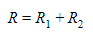When the resistors are connected in parallel, the equivalent resistance is less than the value of either of the two resistors. The potential difference across the resistors R1 and R2 is the same. The current is divided between the paths of the resistors and thus more paths are available for the charge to pass through. Hence, the current is higher.Figure 4: Resistance in Parallel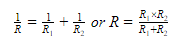Cells in Series Connection

In a battery, if the positive terminal of a cell is connected to a negative terminal of another cell, as illustrated in Figure 5, the cells are said to be connected in series. The overall potential difference across the battery is the algebraic sum of the potential difference of the individual cells.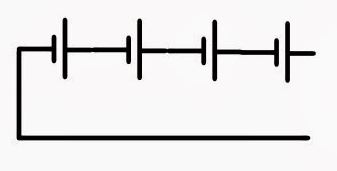Figure 5: Cells in Series

Cells in Parallel Connection

In a battery, if the positive terminals of the cells are connected together and the negative terminal are connected together, as shown in Figure 6, the cells are said to be connected in parallel. The total current delivered by this battery is the algebraic sum of the current discharged by individual cell.Figure 6: Cells in Parallel

Conservation of Charge

The law of conservation of charge states that the total charge in an isolated system never changes. In other words, charges can neither be created nor destroyed. Charges can only be transferred. If a positive charge appears in a system, it is accompanied by the appearance of a negative charge somewhere else in the system. For example: When a plastic rod is rubbed with woollen cloth, the plastic rod is negatively charged and the woollen cloth is positively charged. Thus, the net charge in the system remains the same.

Summary

• Electric current is the rate of flow of a charge.
• Conventional current is a flow carried by electrons, which travel from negative to positive.
• The potential difference across a resistor measures the electrical energy converted per unit of charge passing through the resistor.
• The equivalent resistance of resistors in series is given by the algebraic sum of the resistances of all the resistors.
• The equivalent resistance of resistors in parallel is given by• Cells can be arranged in series to increase the total potential difference of the battery.
• Cells can be arranged in parallel to increase the total current of the battery.
• The total charge in an isolated system never changes.